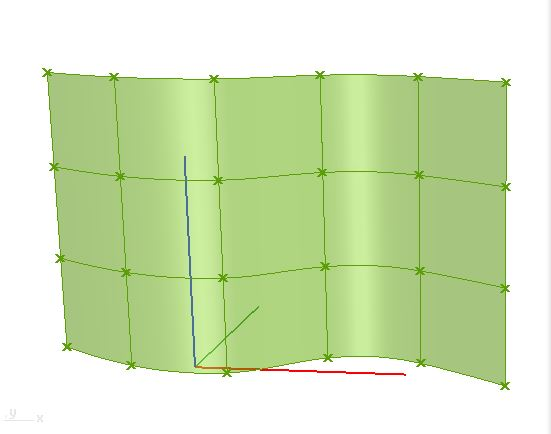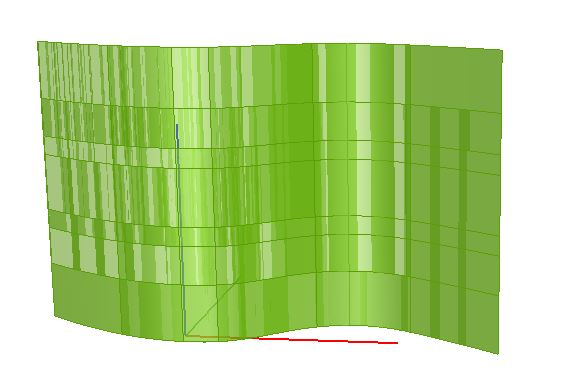# C# Surface.Trim Method

Hello,
I am trying to split the surface with surface.trim method. I tried got the points on U and V direction and want to trim the surface at that location. result shall be similar to first image.
I am bit confused with surface domain and Interval class relation.

attached script and images

``````List<Surface> sfs = new List<Surface>();
for(int i = 0; i < U + 1; i++)
{
//GH_Path gp = new GH_Path(i);
for(int j = 0; j < V + 1; j++)
{
Interval iU = new Interval(x.Domain(0).Length / U * i, x.Domain(1).Length / V * j);
Interval iV = new Interval(x.Domain(0).Length / V * j, x.Domain(1).Length / U * i);
//Interval iU = new Interval(????????
//Interval iV = new Interval(???????
Surface s = x.Trim(iU, iV);